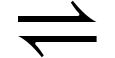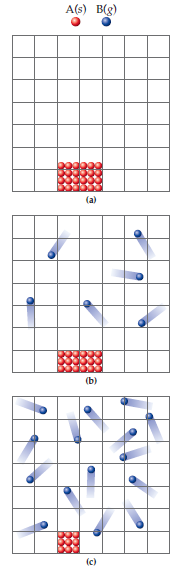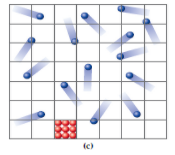×
Get Full Access to Introductory Chemistry - 5 Edition - Chapter 15 - Problem 110p
Get Full Access to Introductory Chemistry - 5 Edition - Chapter 15 - Problem 110p

×

# Solved: Consider the following generic equilibrium in which a solid reactant is inISBN: 9780321910295 34

## Solution for problem 110P Chapter 15

Introductory Chemistry | 5th Edition

• Textbook Solutions
• 2901 Step-by-step solutions solved by professors and subject experts
• Get 24/7 help from StudySoup virtual teaching assistantsIntroductory Chemistry | 5th Edition

4 5 1 308 Reviews
27
5
Problem 110P

Consider the following generic equilibrium in which a solid reactant is in equilibrium with a gaseous product:

A(s)B(g)

These diagrams represent the reaction mixture at the following points: (a) initially; (b) after a short period of time has passed; and (c) at equilibrium.For each diagram, calculate the concentrations of the spheres representing A(s) and B(g). Assume that each block in the grid has an area of 1 cm2 and report your answer in units of spheres/cm2. (Since the spheres in the solid are not free to move, the solid only occupies the area that it covers. The spheres in the solid, however, are free to move and therefore occupy the entire grid.) What do you notice about the concentrations of A(s) and B(g) in these representations? Write an equilibrium expression for the generic reaction and use the results of your calculations to explain why A(s) is not included in the expression.

Step-by-Step Solution:

Step 1 of 3

(1)

Given the area of each grid isand therefore the concentration of both species in each box are;

Box (a)

The total number of A spheres is 24

Since the A is solid. Therefore, the total area occupied will be the actual area enclosed by the sphere.

Hence, the concentration of A;There are no B spheres in box A.

Box (b)

A sphere:

The total number of A spheres is 18.

The number of the boxes they occupy are 2.

Hence, the concentration of A;B sphere:

The total number of B spheres are 6.

The number of boxes they occupy is 49 because they are gaseous molecules and they fill the container completely.

Hence, the concentration of B;Box (c)

A sphere:

The total number of Apheresis 9

The number of the boxes they occupy are 1

Hence, the concentration of A;B sphere:

The total number of B spheres are 15

The number of boxes they occupy is 49

Hence, the concentration of B;Step 2 of 3

Step 3 of 3

##### ISBN: 9780321910295

The answer to “?Consider the following generic equilibrium in which a solid reactant is in equilibrium with a gaseous product:A(s) <math xmlns="http://www.w3.org/1998/Math/MathML"><mo>&#x21CC;</mo>[/itex] B(g)These diagrams represent the reaction mixture at the following points: (a) initially; (b) after a short period of time has passed; and (c) at equilibrium. For each diagram, calculate the concentrations of the spheres representing A(s) and B(g). Assume that each block in the grid has an area of 1 cm2 and report your answer in units of spheres/cm2. (Since the spheres in the solid are not free to move, the solid only occupies the area that it covers. The spheres in the solid, however, are free to move and therefore occupy the entire grid.) What do you notice about the concentrations of A(s) and B(g) in these representations? Write an equilibrium expression for the generic reaction and use the results of your calculations to explain why A(s) is not included in the expression.” is broken down into a number of easy to follow steps, and 153 words. This full solution covers the following key subjects: Equilibrium, Generic, Gaseous, consider, Product. This expansive textbook survival guide covers 19 chapters, and 2046 solutions. Since the solution to 110P from 15 chapter was answered, more than 343 students have viewed the full step-by-step answer. This textbook survival guide was created for the textbook: Introductory Chemistry, edition: 5. Introductory Chemistry was written by and is associated to the ISBN: 9780321910295. The full step-by-step solution to problem: 110P from chapter: 15 was answered by , our top Chemistry solution expert on 05/06/17, 06:45PM.

Unlock Textbook Solution